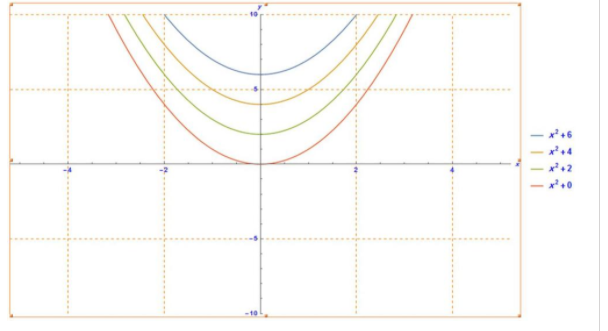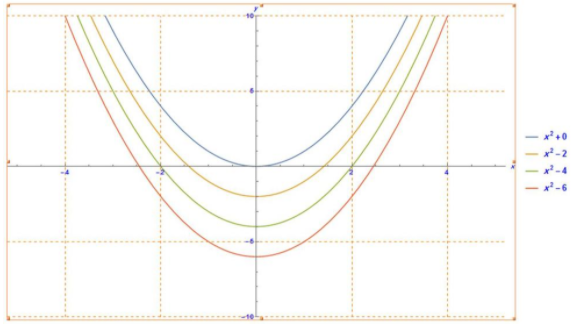# To graph: For the given member of family in viewing rectangle indicated.### Precalculus: Mathematics for Calcu...

6th Edition
Stewart + 5 others
Publisher: Cengage Learning
ISBN: 9780840068071### Precalculus: Mathematics for Calcu...

6th Edition
Stewart + 5 others
Publisher: Cengage Learning
ISBN: 9780840068071

#### Solutions

Chapter 2.2, Problem 72E

(a)

To determine

## To graph: For the given member of family in viewing rectangle indicated.

Expert Solution

### Explanation of Solution

Given information:

The given function is f(x)=cx2 .

The value of c is c=1,12,2,4;[5,5]by[10,10] .

Graph:

The graph for the given family of equations is shown in figure (1).Figure (1)

Interpretation: Graph for the family of equations of the function f(x)=cx2 is shown in figure (1).

(b)

To determine

Expert Solution

### Explanation of Solution

Given information:

The given function is f(x)=cx2 .

The value of c is c=1,1,12,2;[5,5]by[10,10] .

Graph:

The graph for the given family of equations is shown in figure (2).Figure (2)

Interpretation: Graph for the family of equations of the function f(x)=cx2 is shown in figure (2).

(c)

To determine

### The conclusions from the graphs and effects on the value of c .

Expert Solution

As c increases, the graph of the function appears less flattened.

### Explanation of Solution

Given information:

The given function is f(x)=cx2 .

Calculation:

From the graphs in part (a) and (b), we can say that as c increases, the graph of the function appears less flattened and the value of y axis of the minimum point (vertex point) become more.

Therefore, as c increases, the graph of the function appears less flattened.

### Have a homework question?

Subscribe to bartleby learn! Ask subject matter experts 30 homework questions each month. Plus, you’ll have access to millions of step-by-step textbook answers!